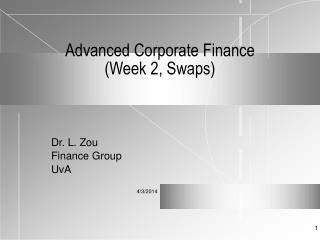DownloadDownload PresentationAdvanced Corporate Finance (Week 2, Swaps)

# Advanced Corporate Finance (Week 2, Swaps)

Télécharger la présentation## Advanced Corporate Finance (Week 2, Swaps)

- - - - - - - - - - - - - - - - - - - - - - - - - - - E N D - - - - - - - - - - - - - - - - - - - - - - - - - - -
##### Presentation Transcript

1. Advanced Corporate Finance (Week 2, Swaps) Dr. L. Zou Finance Group UvA

2. A. INTEREST RATE SWAPS • Interest Rate Swap: Financial agreement to exchange interest payments of a fixed-rate loan with a variable-rate loan. • London Interbank Offer Rate (LIBOR) as a benchmark for the floating rate. • A simple swap contract can be analyzed as two different bonds. • Swap contracts (if default-free) can also be related to a package of forward contracts.

3. Example Firm A and firm B are offered the following terms: Fixed Floating Firm A R(a) = 10.0% r(a) = LIBOR + 0.3% Firm B R(b) = 11.2% r(b) = LIBOR + 1.0% Credit Spread R(b) - R(a) =1.2% r(b) - r(a) = 0.7% • In general, if the credit spreads are not the same, there are potential swap opportunities. • Assume Firm A prefers a floating loan and Firm B wants a fixed loan, and note that R(b)-R(a) > r(b)-r(a).

4. Interest Rate Swap

5. Interest Rate Swap with Financial Intermediary C

6. B. VALUATION OF INTEREST-RATE SWAPS Define B(1) = Value of fixed-rate loan B(2) = Value of floating-rate loan V = B(1) - B(2) = Value of swap Q = Face value of loan k = fixed-rate payment per period = floating-rate payment for time t(i) k* = next floating-rate payment r(i) = continuously compounded discount rate for t(i)

7. Cash flows of a swap with n periods remaining life: t(1) t(2) t(3) … t(n) … (1) - k (2) - k (3) - k (n) - k Following the bond pricing formula And, nothing that a floating-rate bond will sell for its par value if the rate is used as the discount rate,

8. Example A swap involves a 6 month LIBOR floating rate and and 8% fixed rate (semi-annual compounding). It has Q=100,000,000 and a remaining life of 1.25 years. We have t(1)=0.25, t(2)=0.75 and t(3)=1.25. Assume that the appropriate discount rates (continuously compounded) are r(1) = 10%, r(2) = 10.5% and r(3) = 11%; and the last LIBOR = 10.2%. What is the swap’s value?

9. Answer so that The floating-rate payer has lost \$4.27 million in this swap.

10. C. CURRENCY SWAPS • Agreement to exchange both the principal and interest payments an a loan in one currency with that of another currency. • We focus on fixed-rate loans only. • Example: Firm A and firm B are offered the following fixed-rate terms Assume Firm A wants to borrow sterling and firm B wants to borrow dollars.

11. Currency Swap with Financial Intermediary C:

12. D. VALUATION OF CURRENCY SWAPS where S is the exchange rate. Example: See Hull’s book.

13. E. ISSUES AND FACTS CONCERNING SWAPS • Quality spreads tend to increase with loan maturity. • Low credit-worthy party tends to prefer fixed rate loans. • Credit spread differential persists over time and “credit arbitrage”do not eliminate these differentials. • There are default and market riskes involved in swaps. • The potential ‘systematic risk” of swaps, i.e., the chain-effects upon default of a party, is still not well understood.